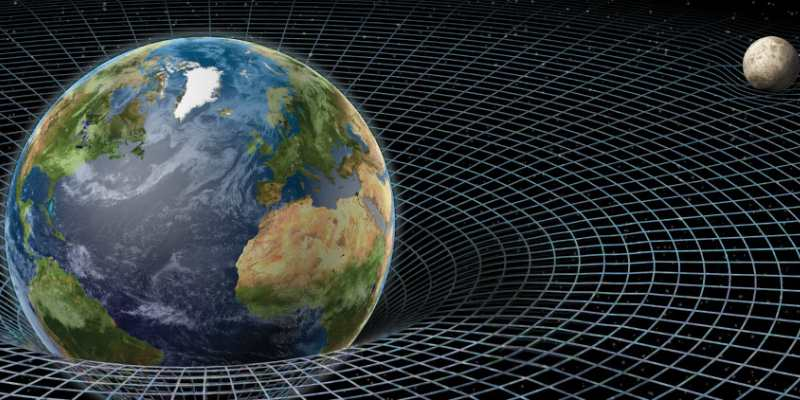### Gravitation Quiz Questions and AnswersAccording to the universal law of gravitation, the force of attraction between bodies is directly proportional to the product of their masses is inversely proportional to the square of the distance between them. Gravitational force is a central force and conservative in nature. So take this quiz and know about gravitation.

#### 1. Who gives the universal law of gravitation?

 56930 Einstein 56931 Sir Isaac Newton 56932 Both of the above 56933 None of the above

#### 2. An object is said to be......... When it is falling freely under the action of gravity.

 56934 Heavy 56935 Light 56936 Weightless 56937 None of the above

#### 3. Acceleration due to gravity at poles is...... Then at the equator.

 56938 Smaller 56939 Equal 56940 Greater 56941 All of the above

#### 4. The minimum velocity required to through a body away from the surface of the earth so that it may not return is called...,...

 56942 Final velocity 56943 Initial velocity 56944 Escape velocity 56945 Orbital velocity

#### 5. The force of gravitation between two bodies does not depend on...

 56946 Their separation 56947 Product of their masses 56948 The sum of their masses 56949 The gravitational constant

#### 6. The acceleration due to gravity...

 56950 Has the same value everywhere in space 56951 Has the same value everywhere on a the earth 56952 Varies with the latitude on the earth 56953 Is Greater on the Moon due to its smaller diameter

#### 7. When an object is thrown up, the force of gravity.......

 56954 Is opposite to the direction of motion 56955 Is in the same direction as the direction of motion 56956 Decreases as it Rises up 56957 Increases as it Rises up

#### 8. The escape velocity of an object from the surface of the earth is 11.6 km/s. If the mass of the earth is increased four times, the resultant escape velocity would be.....

 56958 11.6 km/s 56959 11.6*2 km/s 56960 11.6*4 km/s 56961 11.6*1/2 km/s